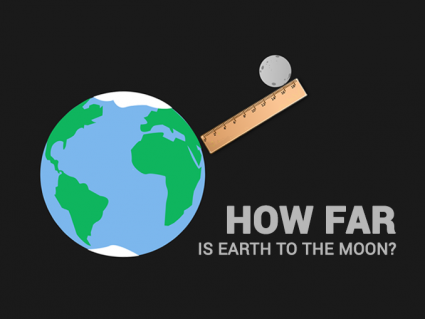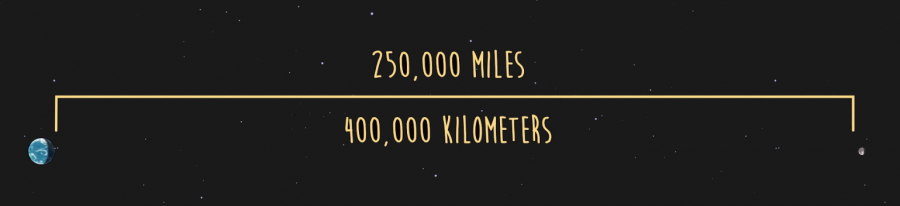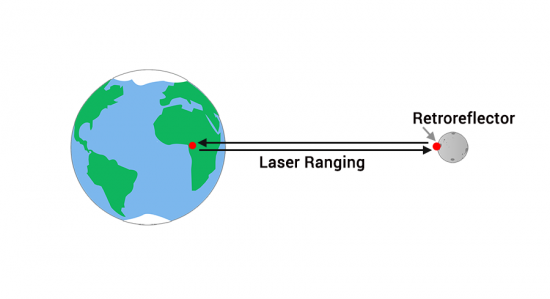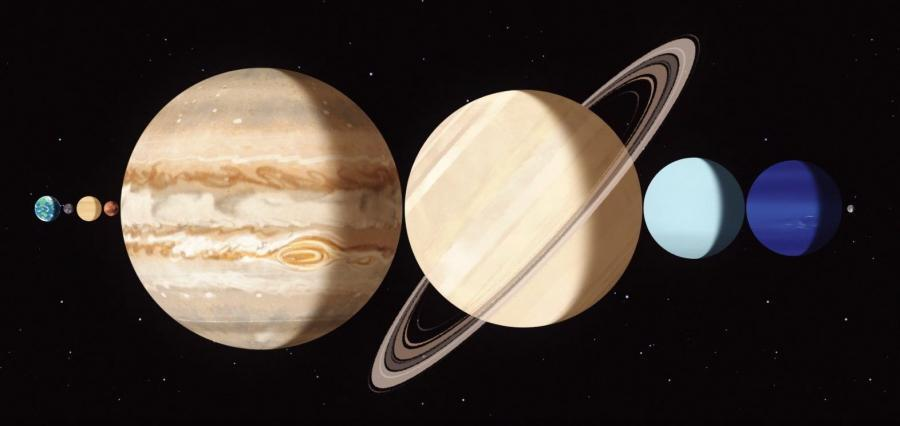# How Far Is the Moon?If only there was a ruler long enough to reach the moon…

The moon orbits the Earth. But it has an elliptical orbit so it’s not a constant distance away.

If you take an average distance, the moon is approximately 238,855 miles (384,400 km) away from the Earth.

So, it varies between 225,622 miles and 252,088 miles due to its elliptical orbit.

This distance is equivalent to the “lunar distance” measurement.### How do we know how far away the moon is?Astronauts from 3 of the Apollo missions placed special reflectors on the lunar surface.

Using these reflectors, we can determine with extreme precision how far away the moon is.

In fact, we know the moon is ever so slightly moving away from the Earth by about an inch and a half per year.

### How far is the moon?

The moon is about one-quarter of 1 million miles away from Earth.

It’s such a big gap that you can just about fit all the 7 other planets in our solar system in between.### 1 thought on “How Far Is the Moon?”

1.two lasers on the poles, and measuring the angle to the iss reflector then the distance from the pole to pole, and the same for the equators and should give the exact distance of the curvature of the earth. a radar refection, and laser, is too distant to measure